Next: Determination of Phase-Shifts Up: Scattering Theory Previous: Partial Waves

# Optical Theorem

The differential scattering cross-sectionis simply the modulus squared of the scattering amplitude. The total cross-section is given by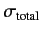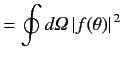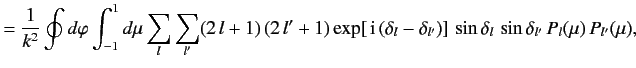(980)

where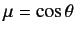. It follows that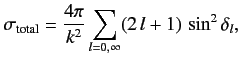(981)

where use has been made of Equation (967). A comparison of this result with Equation (979) yields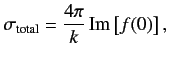(982)

since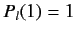. This result is known as the optical theorem. It is a reflection of the fact that the very existence of scattering requires scattering in the forward (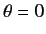) direction in order to interfere with the incident wave, and thereby reduce the probability current in this direction.

It is usual to write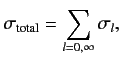(983)

where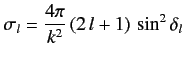(984)

is theth partial cross-section: i.e., the contribution to the total cross-section from theth partial wave. Note that the maximum value for theth partial cross-section occurs when the phase-shift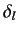takes the value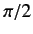.Next: Determination of Phase-Shifts Up: Scattering Theory Previous: Partial Waves
Richard Fitzpatrick 2013-04-08